# Gear trains

26. Dec 2017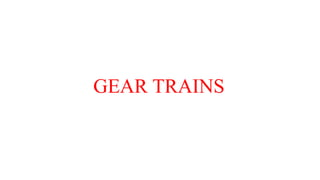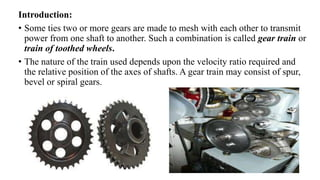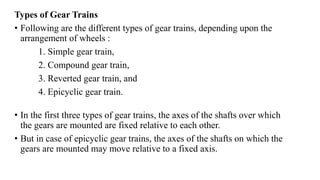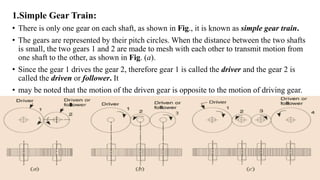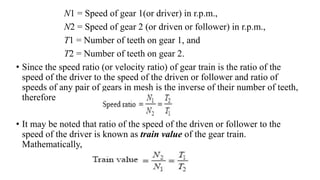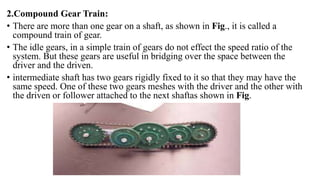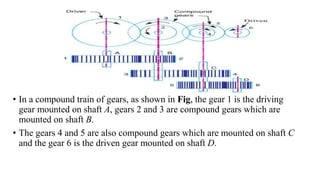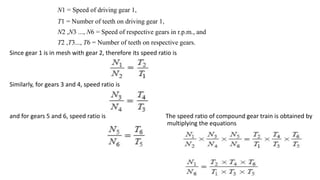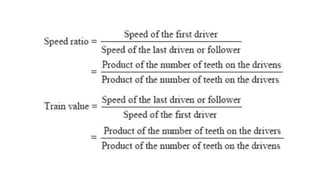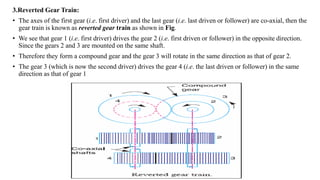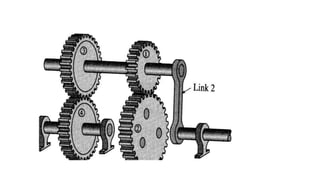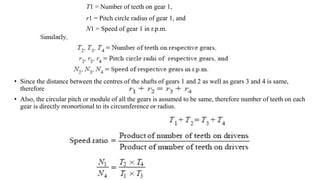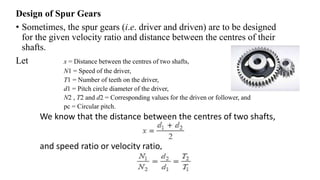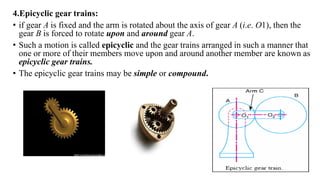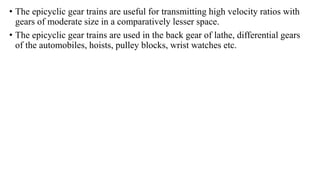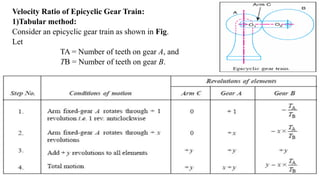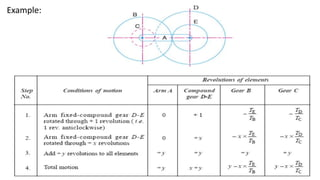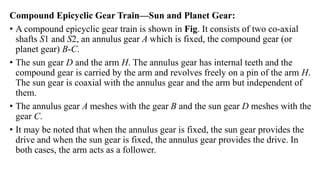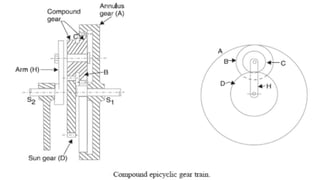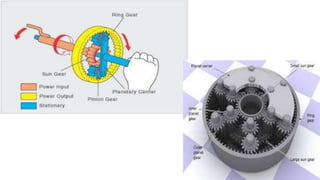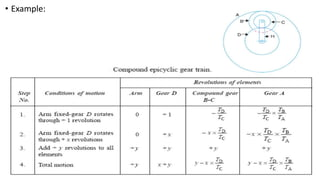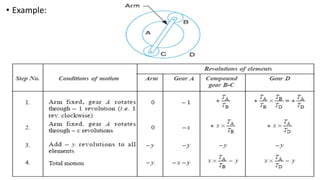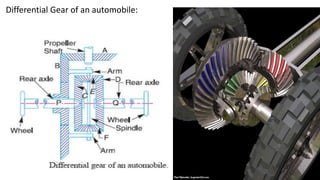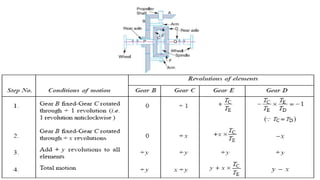1 von 24

### Gear trains

• 1. GEAR TRAINS
• 2. Introduction: • Some ties two or more gears are made to mesh with each other to transmit power from one shaft to another. Such a combination is called gear train or train of toothed wheels. • The nature of the train used depends upon the velocity ratio required and the relative position of the axes of shafts. A gear train may consist of spur, bevel or spiral gears.
• 3. Types of Gear Trains • Following are the different types of gear trains, depending upon the arrangement of wheels : 1. Simple gear train, 2. Compound gear train, 3. Reverted gear train, and 4. Epicyclic gear train. • In the first three types of gear trains, the axes of the shafts over which the gears are mounted are fixed relative to each other. • But in case of epicyclic gear trains, the axes of the shafts on which the gears are mounted may move relative to a fixed axis.
• 4. 1.Simple Gear Train: • There is only one gear on each shaft, as shown in Fig., it is known as simple gear train. • The gears are represented by their pitch circles. When the distance between the two shafts is small, the two gears 1 and 2 are made to mesh with each other to transmit motion from one shaft to the other, as shown in Fig. (a). • Since the gear 1 drives the gear 2, therefore gear 1 is called the driver and the gear 2 is called the driven or follower. It • may be noted that the motion of the driven gear is opposite to the motion of driving gear.
• 5. N1 = Speed of gear 1(or driver) in r.p.m., N2 = Speed of gear 2 (or driven or follower) in r.p.m., T1 = Number of teeth on gear 1, and T2 = Number of teeth on gear 2. • Since the speed ratio (or velocity ratio) of gear train is the ratio of the speed of the driver to the speed of the driven or follower and ratio of speeds of any pair of gears in mesh is the inverse of their number of teeth, therefore • It may be noted that ratio of the speed of the driven or follower to the speed of the driver is known as train value of the gear train. Mathematically,
• 6. 2.Compound Gear Train: • There are more than one gear on a shaft, as shown in Fig., it is called a compound train of gear. • The idle gears, in a simple train of gears do not effect the speed ratio of the system. But these gears are useful in bridging over the space between the driver and the driven. • intermediate shaft has two gears rigidly fixed to it so that they may have the same speed. One of these two gears meshes with the driver and the other with the driven or follower attached to the next shaftas shown in Fig.
• 7. • In a compound train of gears, as shown in Fig, the gear 1 is the driving gear mounted on shaft A, gears 2 and 3 are compound gears which are mounted on shaft B. • The gears 4 and 5 are also compound gears which are mounted on shaft C and the gear 6 is the driven gear mounted on shaft D.
• 8. N1 = Speed of driving gear 1, T1 = Number of teeth on driving gear 1, N2 ,N3 ..., N6 = Speed of respective gears in r.p.m., and T2 ,T3..., T6 = Number of teeth on respective gears. Since gear 1 is in mesh with gear 2, therefore its speed ratio is Similarly, for gears 3 and 4, speed ratio is and for gears 5 and 6, speed ratio is The speed ratio of compound gear train is obtained by multiplying the equations
• 10. 3.Reverted Gear Train: • The axes of the first gear (i.e. first driver) and the last gear (i.e. last driven or follower) are co-axial, then the gear train is known as reverted gear train as shown in Fig. • We see that gear 1 (i.e. first driver) drives the gear 2 (i.e. first driven or follower) in the opposite direction. Since the gears 2 and 3 are mounted on the same shaft. • Therefore they form a compound gear and the gear 3 will rotate in the same direction as that of gear 2. • The gear 3 (which is now the second driver) drives the gear 4 (i.e. the last driven or follower) in the same direction as that of gear 1
• 12. T1 = Number of teeth on gear 1, r1 = Pitch circle radius of gear 1, and N1 = Speed of gear 1 in r.p.m. • Since the distance between the centres of the shafts of gears 1 and 2 as well as gears 3 and 4 is same, therefore • Also, the circular pitch or module of all the gears is assumed to be same, therefore number of teeth on each gear is directly proportional to its circumference or radius.
• 13. Design of Spur Gears • Sometimes, the spur gears (i.e. driver and driven) are to be designed for the given velocity ratio and distance between the centres of their shafts. Let x = Distance between the centres of two shafts, N1 = Speed of the driver, T1 = Number of teeth on the driver, d1 = Pitch circle diameter of the driver, N2 , T2 and d2 = Corresponding values for the driven or follower, and pc = Circular pitch. We know that the distance between the centres of two shafts, and speed ratio or velocity ratio,
• 14. 4.Epicyclic gear trains: • if gear A is fixed and the arm is rotated about the axis of gear A (i.e. O1), then the gear B is forced to rotate upon and around gear A. • Such a motion is called epicyclic and the gear trains arranged in such a manner that one or more of their members move upon and around another member are known as epicyclic gear trains. • The epicyclic gear trains may be simple or compound.
• 15. • The epicyclic gear trains are useful for transmitting high velocity ratios with gears of moderate size in a comparatively lesser space. • The epicyclic gear trains are used in the back gear of lathe, differential gears of the automobiles, hoists, pulley blocks, wrist watches etc.
• 16. Velocity Ratio of Epicyclic Gear Train: 1)Tabular method: Consider an epicyclic gear train as shown in Fig. Let TA = Number of teeth on gear A, and TB = Number of teeth on gear B.
• 17. Example:
• 18. Compound Epicyclic Gear Train—Sun and Planet Gear: • A compound epicyclic gear train is shown in Fig. It consists of two co-axial shafts S1 and S2, an annulus gear A which is fixed, the compound gear (or planet gear) B-C. • The sun gear D and the arm H. The annulus gear has internal teeth and the compound gear is carried by the arm and revolves freely on a pin of the arm H. The sun gear is coaxial with the annulus gear and the arm but independent of them. • The annulus gear A meshes with the gear B and the sun gear D meshes with the gear C. • It may be noted that when the annulus gear is fixed, the sun gear provides the drive and when the sun gear is fixed, the annulus gear provides the drive. In both cases, the arm acts as a follower.
• 21. • Example:
• 22. • Example:
• 23. Differential Gear of an automobile: